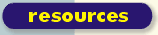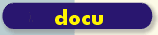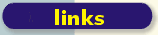scsh.net comp.lang.scheme.scsh 1999 ``` From: Olin Shivers Newsgroups: comp.lang.scheme.scsh Subject: Rewriting Dominicus' script Date: 02 Aug 1999 19:12:14 -0400 Organization: Artificial Intelligence Lab, MIT Message-ID: Friedrich Dominicus had the following awk script, which he wanted to rewrite as a scsh script: df | awk 'BEGIN{sum=0;free=0}\ {if (NR > 1) {sum = sum+\$3; free=free+\$4}}\ END{print "Es sind", (sum/1024), "MB belegt."; print "Und noch", (free/1024), "MB frei"}' It runs df, and adds up the free and used disk space. He wrote it in scsh as follows: ------------------------------------------------------------------------------- #!/usr/bin/scsh \ -e main -s !# (define (df-free) (let ((df-out (run/port (df))) (read-df-out (field-reader (infix-splitter)))) (awk (read-df-out df-out) (record fields) line-number ((sum-free 0) (sum-occ 0)) ((> line-number 1) (values (+ sum-free (string->number (nth fields 3))) (+ sum-occ (string->number (nth fields 2))))) (after (values (/ sum-free 1024.0) (/ sum-occ 1024.0)))))) (define (main argv) (receive (sum-free sum-occ) (df-free) (format #t "Es sind noch ~a MB frei und ~a MB belegt~%" sum-free sum-occ))) ------------------------------------------------------------------------------- This is basically right. We can improve it in some small ways: - The AWK macro isn't indented perfectly; - we can punt the LINE-NUMBER var and the (> LINE-NUMBER 1) test by simply reading in a line before executing the AWK form; - I changed the variable names a bit & added a line or two of documentation. - I made df-free clean up after itself by closing the pipe that connects it to the df process. Not super important here, since this process will exit immediately afterwards (and gc will always clean up after us, anyway), but if we are going to encapsulate the code in a procedure, make the procedure useful in as broad a context as possible. - I simplified (FIELD-READER (INFIX-SPLITTER)) to just (FIELD-READER). - I added a single-sentence comment at the beginning, since I hate shell scripts that make you puzzle out what they do. One sentence saves you a lot of time. This gives us the following script. I wrote the core procedure two different ways -- once with awk, and once with an explicit loop (since we aren't really using many of the features of awk much). It's about equally good either way, I think. (And see REDUCE-PORT for a third way to do it.) ------------------------------------------------------------------------------- #!/usr/local/bin/scsh \ -e main -s !# ;;; Add up the number of kb of free and used disk space for all the volumes ;;; reported by df(1). (define (df-used+free) (let ((df-out (run/port (df))) (df-read (field-reader))) (read-line df-out) ; Skip the first line from df. (let lp ((free-kb 0) (used-kb 0)) (receive (line fields) (df-read df-out) (cond ((eof? line) (close df-read) (values (/ free-kb 1024.0) (/ used-kb 1024.0))) (else (loop (+ free-kb (string->number (nth fields 2))) (+ used-kb (string->number (nth fields 3)))))))))) ;;; Same procedure, written with the AWK macro (define (df-used+free) (let ((df-out (run/port (df))) (df-read (field-reader))) (read-line df-out) ; Skip the first line from df. (awk (df-read df-out) (line fields) ((free-kb 0) (used-kb 0)) (else (values (+ free-kb (string->number (nth fields 2))) (+ used-kb (string->number (nth fields 3))))) (after (close df-read) (values (/ free-kb 1024.0) (/ used-kb 1024.0)))))) (define (main argv) (receive (free used) (df-used+free) (format #t "Es sind noch ~a MB frei und ~a MB belegt~%" free used))) ------------------------------------------------------------------------------- Note that Friedrich wrote his *script* as a *program* with a MAIN entry point. There's no big payoff for doing things this way for this script, since the core routine DF-USED+FREE doesn't really capture any terrifically useful functionality we'd want to use from other code. So we could just rewrite it is as a plain script. This saves a few lines of code, since we can punt the DEFINE's and use forms that work on the current input & output ports, etc. I also moved the definition of the record reader to a separate define for no particular reason, though it looks slightly easier to read to my eye. Here's my rewritten script. It forks off the df and pipes the result into the current process as stdin. I punted the port-close operation in the AFTER clause, since the code is now explicitly committed to being a script that exits immediately thereafter. I also, just for fun, changed the output report to be a legitimate s-expression alist -- that is, it no longer prints out Es sind noch 472 MB frei und 314 MB belegt but rather prints ((frei-mb 472) (belegt-mb 314)) If you get in the habit of printing out reports as s-expressions, it's easier to get programs you write later to consume the data. Or get emacs to indent them. Etc. When you use s-expressions for I/O formats, you can parse them much more reliably using READ than you can using all-too-frequently-heuristic parsers based on regexps. ------------------------------------------------------------------------------- #!/usr/local/bin/scsh -s !# ;;; Add up the number of kb of free and used disk space for all the volumes ;;; reported by df(1). (define df-read (field-reader)) (exec-epf (| (df) (begin (read-line) ; Skip the first line from df. (awk (df-read) (line fields) ((free-kb 0) (used-kb 0)) (else (values (+ free-kb (string->number (nth fields 2))) (+ used-kb (string->number (nth fields 3))))) (after (format #t "((frei-mb ~a) (belegt-mb ~a))\n" (/ free-kb 1024.0) (/ used-kb 1024.0))))))) ------------------------------------------------------------------------------- By the way, advanced scsh hackers will note that we could have done the fork-off-a-df-and-feed-us-the-input-on-stdin hack procedurally, without using the process forms, as follows: (fork/pipe (lambda () (exec "df"))) (read-line) ; Skip the first line from df. (awk (df-read) (line fields) ((free-kb 0) (used-kb 0)) (else (values (+ free-kb (string->number (nth fields 2))) (+ used-kb (string->number (nth fields 3))))) (after (format #t "((frei-mb ~a) (belegt-mb ~a))\n" (/ free-kb 1024.0) (/ used-kb 1024.0))))) But it doesn't really matter much. We aren't pushing the notation hard either way, so they are both fairly clear. In any event, perhaps this will go to show that perl is not the only language where "there's more than one way to do it.". -Olin ``` Up